MathematicsExample: Solving a Fully Implicit ODE Problem

The following example shows how to use the function `ode15i` to solve the implicit ODE problem defined by Weissinger's equation

•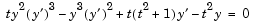with the initial value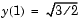. The exact solution of the ODE is

•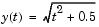The example uses the function `weissinger`, which is provided with MATLAB, to compute the left-hand side of the equation.

Before calling `ode15i`, the example uses a helper function `decic` to compute a consistent initial value for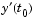. In the following call, the given initial value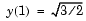is held fixed and a guess of 0 is specified for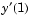. See the reference page for `decic` for more information.

• ```t0 = 1;
y0 = sqrt(3/2);
yp0 = 0;
[y0,yp0] = decic(@weissinger,t0,y0,1,yp0,0);
```

You can now call `ode15i` to solve the ODE and then plot the numerical solution against the analytical solution with the following commands.

• ```[t,y] = ode15i(@weissinger,[1 10],y0,yp0);
ytrue = sqrt(t.^2 + 0.5);
plot(t,y,t,ytrue,'o');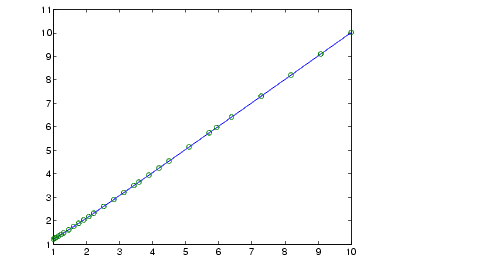```Solver for Fully Implicit ODEs Changing ODE Integration Properties© 1994-2005 The MathWorks, Inc.